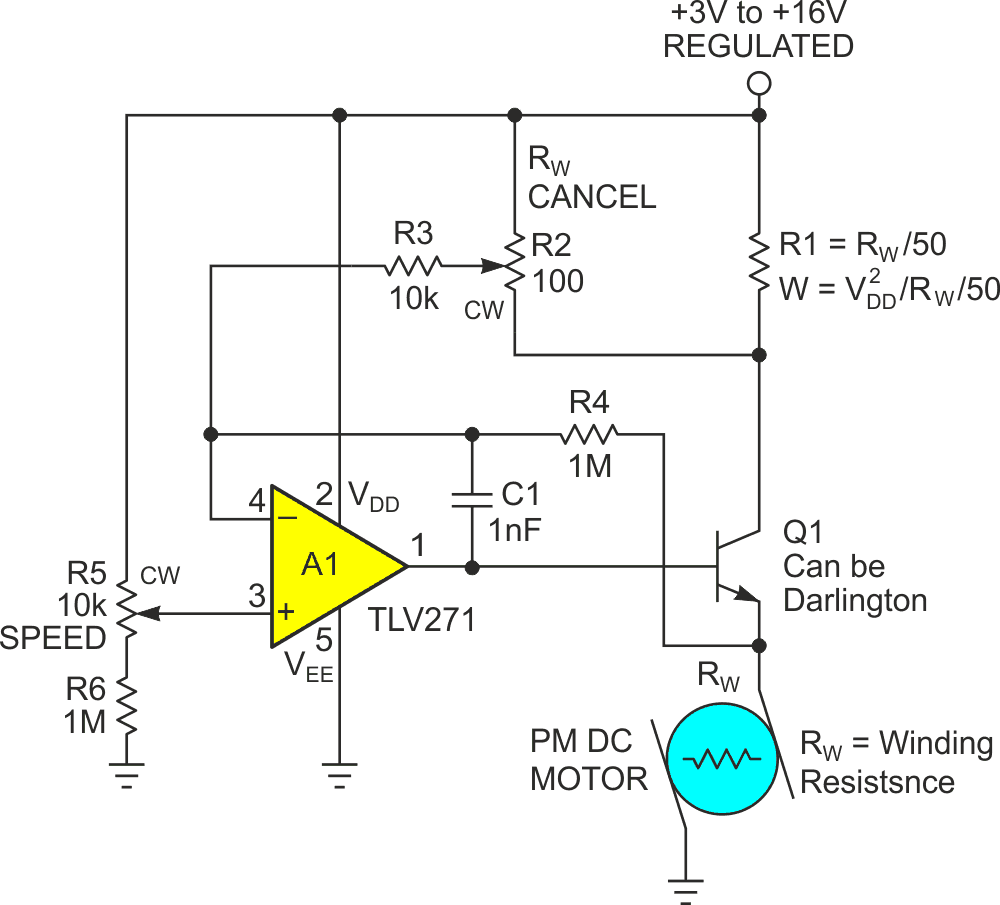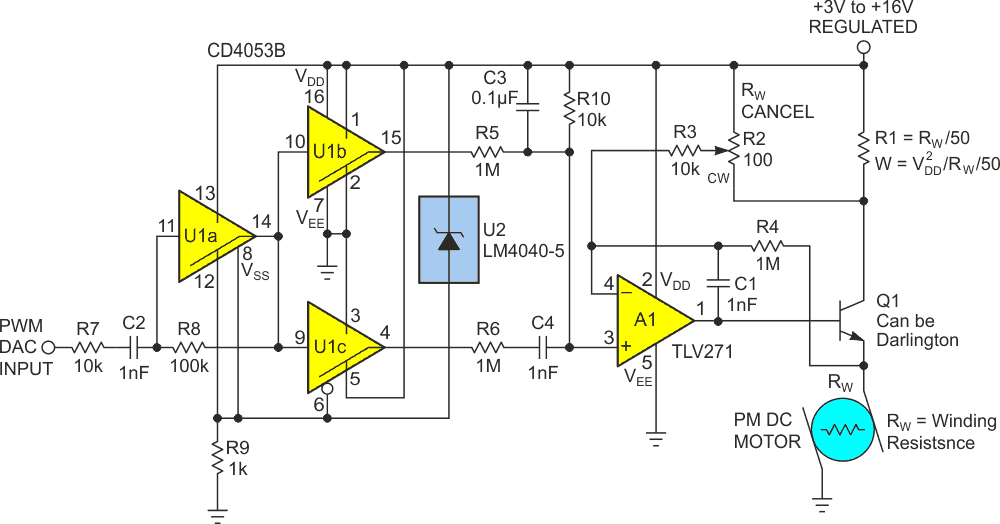# Precision DC motor speed control via pot or DAC

## Texas Instruments CD4053B

Ideally (in that magical land where theory and practice are the same thing), the speed of a permanent magnet DC motor is exactly proportional to the input voltage: Speed = KSV, where constant KS is specific to the motor in question. But since real motors exist alongside us in the practical world, their behavior differs from this theoretical ideal, mostly because real motors include resistance: Mechanical resistance (friction), and electrical (winding resistance = RW). To produce the torque needed to overcome the former, motors must draw current (I) and when they do, current passing through RW decreases the effective driving voltage (–IRW). Therefore, when a motor is loaded by internal and/or external friction, current draw increases and effective voltage decreases. So, it slows down, as predicted by:

S = KS(V – I•RW).

One way to reduce (or eliminate) this effect and make motor speed constant, despite varying frictional loading, is to actively cancel RW by sensing current I and adding a proportional compensating term to V, thereby forcing:

S = KS(V – I•RW + I•RW) = KSV.

Figure 1 presents a simple, versatile circuit that does exactly that, plus the convenience of grounding one of the motor connections. Here’s how it works.Figure 1. The RW-cancelling motor drive.

Rail-to-rail op-amp A1 compares the speed setpoint from potentiometer R5, variable from (0.99)VDD to VDD, to the motor drive voltage VS that is output by Q1 via the 100:1 R4/R3 voltage divider feedback loop, and servos Q1 base current accordingly. VS is thereby settable from a minimum of zero (R5 fully CCW), to a maximum of VDD minus the sum of the saturation voltages of A1 and Q1 (fully CW). The usable limits of VDD are 3 V to 16 V, corresponding with A1’s TLV271 datasheet ratings. The VDD and Q1 should be chosen for compatibility with motor drive (VS and current) requirements.

Motor current I, as reflected in Q1’s collector current, is sensed by R1. An adequately accurate estimate for R1, given that cancellation is ultimately fine-tuned with R2, can be based on a simple multimeter measurement of RW while the motor shaft is held stationary. Typical R1 resistances (R1 = RW/50) will lie in the milliohm range, making implementation of R1 with a simple circuit-board-trace meander feasible. This is a good thing because a circuit board trace, being copper, will have a temperature coefficient similar to the (likewise copper) motor wiring: ~3930 ppm/°C, which will improve the stability of RW cancellation versus temperature, provided that motor and R1 are in similar thermal environments. Note that R1’s voltage drop due to its connection to Q1’s collector isn’t subtracted from max motor drive, but instead makes due with the (otherwise wasted) difference between Q1’s base and collector saturation voltages. Note that the wattage rating needed for R1 = VDD2/RW/50 and a multiple of the IR1 voltage developed (as set by R2) is added to VS via the +40 dB R4/R3 feedback loop.

Proper adjustment of R2 for RW cancellation and constant speed independent of friction will thereby result in the (magical?):

KS(V – I•RW + I•RW) = KSV.

R2 adjustment can be accomplished in a variety of ways. For example, motor speed can be quantitatively measured (e.g., with an inexpensive optical tachometer) where R2 is adjusted for a constant speed while frictional load is varied. Or it can be done subjectively (by ear, listening to the pitch of the spinning motor’s whine) for a similarly accurate result – if you can read music.

The Figure 1 circuit can thus generate a dialed-in motor drive voltage and compensate it for winding resistance, thereby achieving, and holding an accurate and stable set speed. But that speed is manually set by a pot wiper position. What if we need a more automated source of motor control?

Figure 2 answers this question with a variant of Figure 1 that replaces potentiometer R5 with an inexpensive ripple-canceled PWM-DAC.Figure 2. PWM DAC control of RW-cancelling motor drive.

Gate U1a of triple-switch U1 regeneratively translates the logic level PWM input at R7 into a square wave output for gates U1b and U1c. VDD voltages between 3 and 5 V pass directly to U1 pin 8 (VSS) via R9, while VDD > 5 V causes shunt regulator U2 to kick in, limiting U1’s VDD – VSS to a logic compatible 5 V.

U1b generates an uninverted PWM waveform for low-pass averaging by the R5, C3, and R10 network and output of VS, while U1c cranks out an AC-coupled inverted version suitable for analog subtraction ripple cancellation via R6C4 per the scheme described in this design idea .

EDN